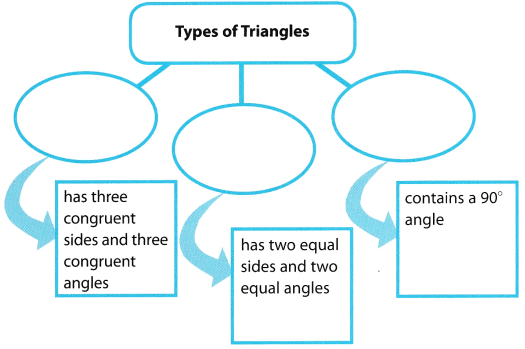# Texas Go Math Grade 6 Module 16 Answer Key Area and Volume Equations

Refer to our Texas Go Math Grade 6 Answer Key Pdf to score good marks in the exams. Test yourself by practicing the problems from Texas Go Math Grade 6 Module 16 Answer Key Area and Volume Equations.

## Texas Go Math Grade 6 Module 16 Answer Key Area and Volume Equations

Evaluate.

Question 1.
$$\frac{1}{2}$$(3)(5 + 7)
Solution to this example is given below
$$\frac{1}{2}$$(3)(5 + 7) = $$\frac{1}{2}$$(3)(12) Perform operations inside parentheses first
= 1.5(12) Multiply left to right
= 18 Multiply again.
= 18 Final solution.

Question 2.
$$\frac{1}{2}$$(15)(13 + 17)
Solution to this example is given below
$$\frac{1}{2}$$(15)(13 + 17) = $$\frac{1}{2}$$(15)(30) Perform operations inside parentheses first
= 7.5(30) Multiply left to right
= 225 Multiply again.
= 225 Final solution.

Question 3.
$$\frac{1}{2}$$(10)(9.4 + 3.6)
Solution to this example is given below
$$\frac{1}{2}$$(10)(9.4 + 3.6) = $$\frac{1}{2}$$(10)(13) Perform operations inside parentheses first
= 5(13) Multiply left to right
= 65 Multiply again.
= 65 Final solution.

Question 4.
$$\frac{1}{2}$$(2.1)(3.5 + 5.7)
Solution to this example is given below
$$\frac{1}{2}$$(2.1)(3.5 + 5.7) = $$\frac{1}{2}$$(2.1)(9.2) Perform operations inside parentheses first
= 1.05(9.2) Multiply left to right
= 9.66 Multiply again.
= 9.66 Final solution.

Find the area of each figure.

Question 5.
a triangle with base 6 in. and height 3 in. _____________
Find the area of the triangle
b = 6 inches h = 3 inches
A = $$\frac{1}{2}$$bh
= $$\frac{1}{2}$$(6 inches)(3 inches) Substitute
= 9 square inches Multiply
= 9 in2

Question 6.
a square with sides of 7.6 m ______________
s = 7.6
Write equation of area of a square:
Area = s2
Substitute values:
Area = 7.62
Evaluate:
Area = 57.76
Area of the given square is 57.76 square meters.

Question 7.
a rectangle with length 3$$\frac{1}{4}$$ ft and width 2$$\frac{1}{2}$$ ft. ____________
Solution to this example is given below
$$3 \frac{1}{4}=\frac{3 \times 4+1}{4}=\frac{13}{4}=3.25$$ Convert to decimal number
$$2 \frac{1}{2}=\frac{2 \times 2+1}{2}=\frac{5}{2}=2.5$$ Convert to decimal number
Find the area of the rectangle.
base = 3.25 ft. and height = 2.5 ft
A = bh Use the formula for the area of a parallelogram.
= 3.25 × 2.5 Substitute for base and height.
= 8.125
The area is 8.125 square feet.

Question 8.
a triangle with base 8.2 cm and height 5.1 cm ____________
Find the area of the triangle.
b = 8.2cm h = 5.1 cm
A = bh
= $$\frac{1}{2}$$(8.2 cm)(5.1 cm) Substitute
= 20.91 square centimeters Multiply
= 20.91 cm2

Visualize Vocabulary

Use the ✓words to complete the graphic. You will put one word in each oval.Understand Vocabulary

Match the term on the left to the correct expression on the right.